# Introduction

The `Tendril` package contains functions designed to compute the x-y coordinates and to build a Tendril plot. Inspired by the notabilia visualization, the Tendril plot was developed to capture the relative effect of different kind of adverse events for two treatments, including temporal aspects, in a single visualization. Specifically, each tendril (branch) in the Tendril plot represents a type of adverse effect, and the direction of the tendril is dictated by on which treatment arm the event is occurring. If an event is occurring on the first of the two specified treatment arms, the tendril bends clockwise (to the right). If an event is occurring on the second of the treatment arms, the tendril bends anti-clockwise (to the left).

``````library(Tendril)

data("TendrilData")

test <- Tendril(mydata = TendrilData,
rotations = Rotations,
AEfreqThreshold = 9,
Tag = "Comment",
Treatments = c("placebo", "active"),
Unique.Subject.Identifier = "subjid",
Terms = "ae",
Treat = "treatment",
StartDay = "day",
SubjList = SubjList,
SubjList.subject = "subjid",
SubjList.treatment = "treatment"
)

plot(test)``````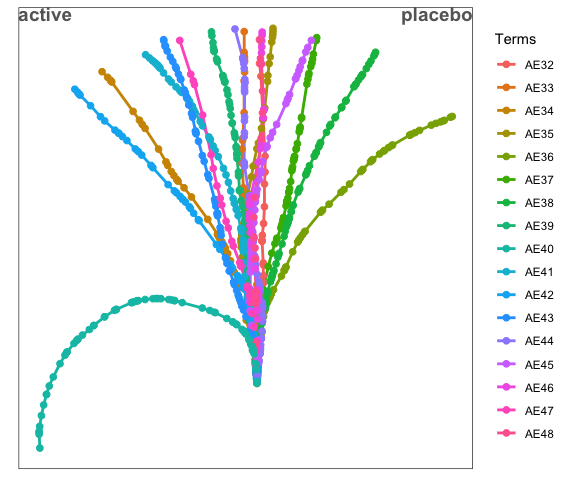``plot(test, coloring = "p.adj")``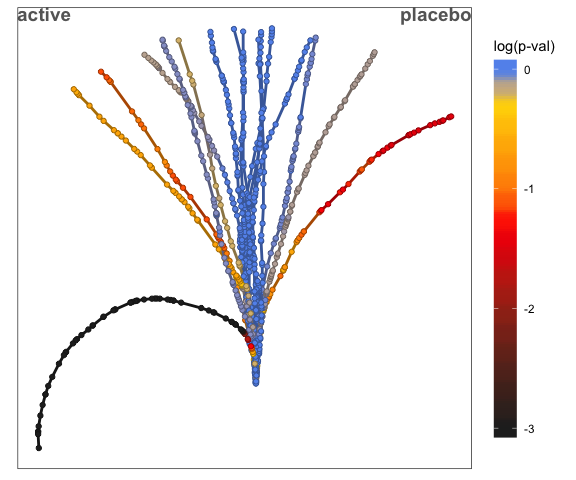``plot(test, term = c("AE40", "AE42", "AE44"))``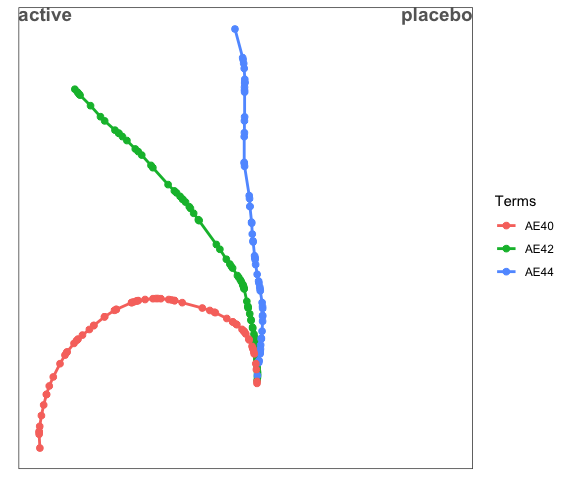In the plots above, a clinical trial with two treatment arms, placebo and active, and 80 different adverse effects were simulated (“AE1” to “AE80”). As outlined above, the Tendril plot is based on an algorithm that evaluates each type of adverse event (AE) in sequence, producing a collection of tendrils (branches) that effectively summarizes the time-resolved safety profile of a clinical trial within a single plot. Events on the first treatment (placebo) cause that tendril to bend clockwise to the right, and each event on the second treatment (active) causes the tendril to bend anti-clockwise to the left. The resulting tree-like structure clearly displays those adverse events having the largest differences in relative risk (see AE40); AEs having only a transient increased risk bending and then straightening (see AE42); and AEs that are balanced over the treatment arms (see AE44). In the first plot each tendril is colored according to adverse event type and in the second, each event has been colored according to the false discovery rate adjusted p value. There are a number of statistical measures that could be used for colouring, see the plot.Tendril documentation.

# Objects within the `Tendril` package

## The `Tendril` class

The result of the `Tendril` function is an object of the class `Tendril` that can be referenced as a base R list. It contains the following elements:

• `data` : a dataframe containing the original data, the calculated angles and coordinates used to produce the tendril plot and the statistical analysis results
• `Terms` : the name of the variable in the source dataset that records the event type (e.g. adverse event)
• `Treat` : the name of the variable in the source dataset that records the treatment
• `Treatments` : the available values of Treatments
• `StartDay` : the name of the variable in the source dataset that records the start day of the adverse event
• `Unique.Subject.Identifier` : the name of the variable in the source dataset that records the subject identifier
• `AEfreqThreshold` : the frequency threshold used to select tendrils
• `Tag` : a text label associated with the analysis
• `n.tot` : a dataframe with a single row and variables for the total number of events recorded for each of the treatments
• `SubjList` : A dataframe listing all the subjects in the trial, including those not having an AE, and corresponding treatments
• `SubjList.subject` : the name of the column in `SubjList` containing the subject IDs
• `SubjList.treatment` : the name of the column in `SubjList` containing the treatments names

## The `TendrilPerm` class

The result of the `TendrilPerm` function is an object of the class `TendrilPerm`. This object can also be referenced as a list with the following elements:

• `tendril` : A `Tendril` object corresponding to the arguments passed to `TendrilPerm`
• `PermTerm` : The event type for which permutations are computed
• `perm.data` : A dataframe recording the coordinates of the permuted tendril data
• `tendril.pi` : An object of class `TendrilPi` recording estimated percentiles on the assumption of balance between treatment arms

## The `TendrilPi` class

The `TendrilPerm` function outputs an object which contains an element of class `TendrilPi`. This is structurally similar to a data frame, with equal length vector elements for event day (`StartDay`), `Terms`, `x` and `y` coordinates, `Tag`, number of terms (`TermsCount`), `label` (whether upper or lower limit), `type` (`"Percentile"`) and the day from which to permute (`perm.from.day`).

# Functions within the `Tendril` package

## `Tendril()`

The `Tendril` function requires several arguments. The key argument is `mydata` a data frame with at least four columns, corresponding to a subject identifier, treatment arm, event type and day (relative to randomisation) of onset. Four character variables are also passed to denote the column name of the required columns; these are `Unique.Subject.Identifier`, `Treat`, `Terms` and `StartDay` respectively. If any additional columns are present then these are retained for subsequent analysis.

Additionally arguments are provided for the unadjusted angular displacement of each event (`rotations`, either a single value for all records or a vector which can vary by row of `mydata`); a minimum value for the number of events in at least one arm (`AEfreqThreshold`); a text label to apply to the analysis as a whole (`Tag`); the two treatments to be compared (`Treatments`, any other treatments are ignored).

A data frame can optionally be passed as the argument `SubjList` which lists all the subjects in the trial, including those not having an AE, and the corresponding treatments to which each subject has been randomised along with (optionally) the day to which each subject was followed up. Even though the SubjList data frame is optional, it is required to calculate statistics and simulate permuted tendrils (described below). Three character arguments (`SubjList.subject`, `SubjList.treatment` and `SubjList.dropoutday`) are then also passed to allow the variables in `SubjList` to be correctly identified.

Finally a number of binary flags can be passed to further control the analysis. `compensate_imbalance_groups` allows for treatment group imbalance to be compensated for, provided `SubjList` is present. `filter_double_events` allows either all, or just the first event of each type to be recorded for each subject. Finally, `suppress_warnings` allows warnings from the Chi-square test to be disabled, as low counts can result in multiple warning messages.

A typical Tendril dataset might look like this:

``````##   subjid treatment   ae day
## 1  ID240   placebo AE40 134
## 2  ID101   placebo AE43 263
## 3  ID101   placebo AE41  44
## 4  ID102   placebo AE37 134
## 5  ID102   placebo AE36  98
## 6  ID102   placebo AE39  50``````

Note the four columns containing the subject IDs (`subjid`), the treatment (`treatment`), the adverse effect term (`ae`) and the days (`day`).

The `Tendril()` function could then be called as:

``````test <- Tendril(mydata = TendrilData,
rotations = Rotations,
AEfreqThreshold = 9,
Tag = "Comment",
Treatments = c("placebo", "active"),
Unique.Subject.Identifier = "subjid",
Terms = "ae",
Treat = "treatment",
StartDay = "day",
SubjList = SubjList,
SubjList.subject = "subjid",
SubjList.treatment = "treatment"
)``````

NB: If there is any missing data in the subject identifier, treatment, event type or onset day then such rows will be removed.

The function checks that the arguments are valid and then computes the angles and coordinates of the x and y points in the tendrils based on the balance of events between treatments with the angular displacement being determined by the argument `rotations`.

The time between consecutive events of each type is proportional to the distance between connected points on the tendril plot. The angular displacement at each point is determined by the excess number of treatments on the first arm (rotating clockwise) or the excess number of treatments on the second arm (rotating anticlockwise) at each point in time.

If the argument `SubjList` provides a data frame of subjects, treatments and optionally drop-out days then `Tendril` calls the function `tendril_stat` to estimate the statistical significance of the imbalance at each data point. Statistical significance is estimated using an unadjusted Chi-square test (`p`), a Chi-square test false discovery rate (FDR) adjusted locally per AE (`p.adj`), or Fisher’s Exact test (`fish`). Additional statistics are provided for the risk difference (`rdiff`), risk ratio (`RR`) and odds ratio (`OR`).

## `TendrilPerm`

The `TendrilPerm` function required an object of class `Tendril` to be passed (as `tendril`) which is the basis of permutations of the treatment assignment. An argument is also supplied with the event type (`PermTerm`) for which permutations are required. All other event types are ignored in the analysis and removed from the results.

Arguments can also be provided to specify the number of permutations (`n.perm`; defaults to 100), the day from which to permute treatments (`perm.from.day`; defaults to 1), the lower proportion to estimate (`pi.low`; defaults to 0.1, i.e. 10th percentile), and the upper proportion to estimate (`pi.high`; defaults to 0.9, i.e. 90th percentile).

As well as calculating permuted tendrils on the basis of randomly permuted treatment assignments (corresponding to a hypothesis of no imbalance between treatment arms) the `TendrilPerm` function also returns tendrils corresponding to the specified percentiles of these permutations. These facilitate comparison with the observed tendril to identify any event types with significant imbalance between treatment arms.

The use of the `perm.from.day` argument can be useful to explore temporal effects, for example where there is a strong imbalance initially, which subsequently resolves, with balanced incidence of events from a certain point in time onward.

The function outputs a list with four elements including the input tendril data filtered for the selected event type, the event type selected, the permuted tendril details, and the percentile details in the form of a `TendrilPi` object.

An example of an invocation of the `TendrilPerm` function, using the `Tendril` object generated above is as follows.

``````perm <- TendrilPerm(test,
"AE40",
n.perm = 500)
perm2 <- TendrilPerm(test,
"AE42",
n.perm = 500,
perm.from.day = 120)``````

## Plot functions

### `plot.Tendril`

This function can be invoked as `plot()` applied to a `Tendril` object. As well as providing a `Tendril` object the user can optionally supply `coloring` and `term` arguments. `coloring` controls how the points on the tendril plot are coloured, and defaults to `Terms` meaning that each event type is coloured differently and a legend provided. Alternatively `p`, `p.adj`, `FDR.tot`, `fish`, `rdiff`, `RR` and `OR` will colour each point on the tendril scale according to the relevant statistic at that specific plot point.

The `term` argument allows the plot to display only specific event types. The default is `NULL` which means that all tendrils are displayed. Alternatively, a single value can be supplied, or a vector of multiple terms. In all cases tendrils are only displayed subject to the `AEfreqThreshold` argument.

The following plots illustrate some sample tendril plots.

``plot(test)````plot(test, coloring = "p.adj")````plot(test, term = "AE40", coloring = "fish")``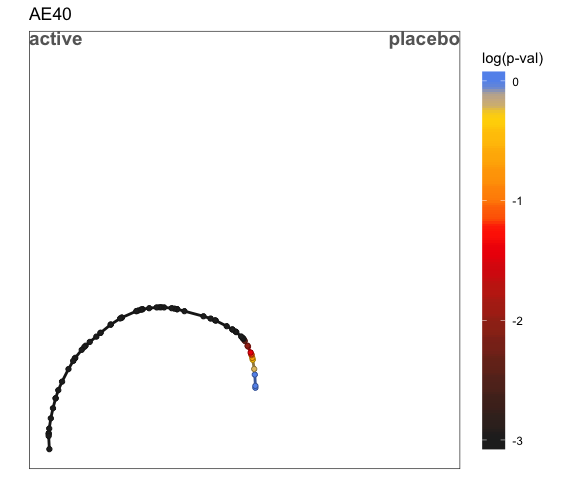``plot(test, term = paste("AE", c(40, 42, 44), sep = ""), coloring = "Terms")``These are generated using the `ggplot2` package and so can be amended using features from the `ggplot2` package.

Tendril plots can also be produced in interactive mode using the `plotly` package. These are requested by passing the optional argument `interactive=TRUE`. These interactive plots allow access to feature such as zooming, and hovering over points to obtain information such as the event type, the FDR p-value and the total number of events of that type.

### `plot.TendrilPerm`

This function is also invoked as `plot`, but applied to a `TendrilPerm` object. As well as passing a `TendrilPerm` object the user can again specify a `coloring` argument. This applies equivalent colouring to that used in `plot.Tendril` but applied only to the selected event type, as defined in the call to `TendrilPerm`. Permuted tendrils are coloured in light grey.

There is also an optional `percentile=TRUE` argument which will overlay the percentiles specified in the call to `TendrilPerm`. These are shown as two dark grey lines.

The following plots illustrate example permutation plots.

``plot(perm)``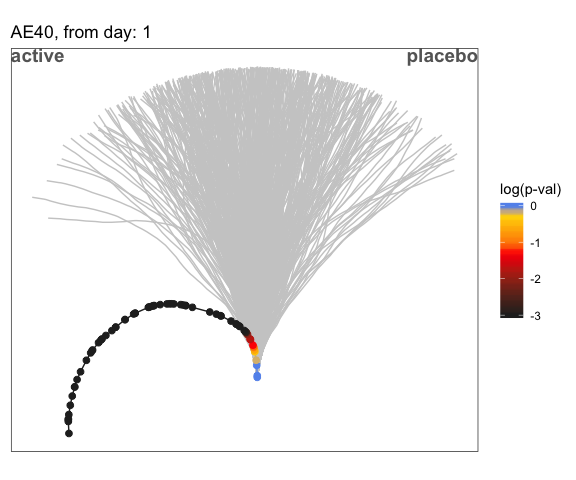``plot(perm, coloring = "OR", percentile = TRUE)``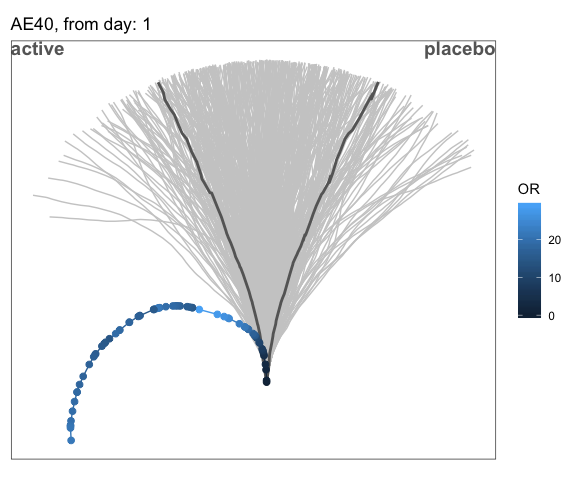``plot(perm2, coloring = "p.adj", percentile = TRUE)``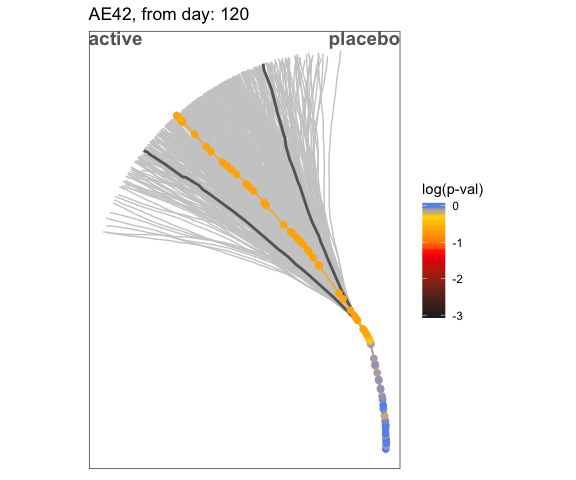Again, these plots are produced using `ggplot2` and can be modified accordingly.

### `plot_timeseries`

This function requires a `Tendril` object to be supplied and optionally a `term` argument, which defaults to `NULL`. The `term` argument operates in an equivalent manner to in the `plot.Tendril` function, and allows specific event types to be selected, unless `NULL` is supplied, in which case all tendrils are displayed.

The `plot_timeseries` function shows the event balance as a linear, rather than radial, plot, with time on the horizontal axis and the event balance on the vertical axis.

Example time series plots are shown below.

``plot_timeseries(test)``